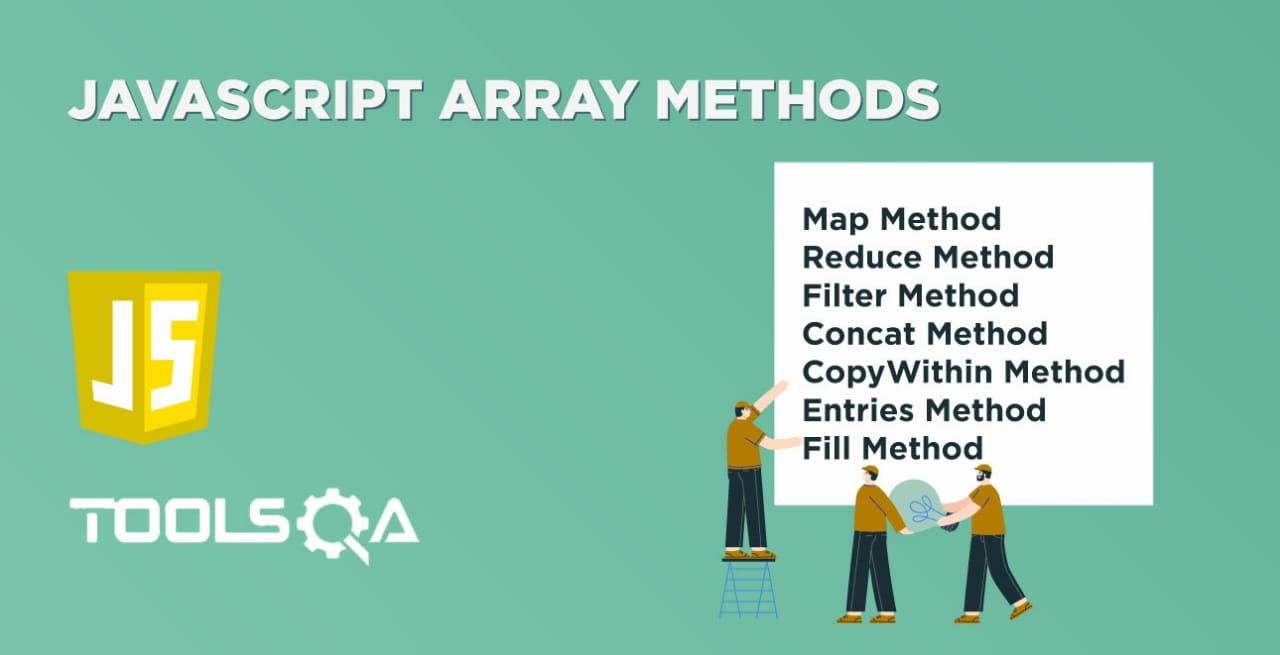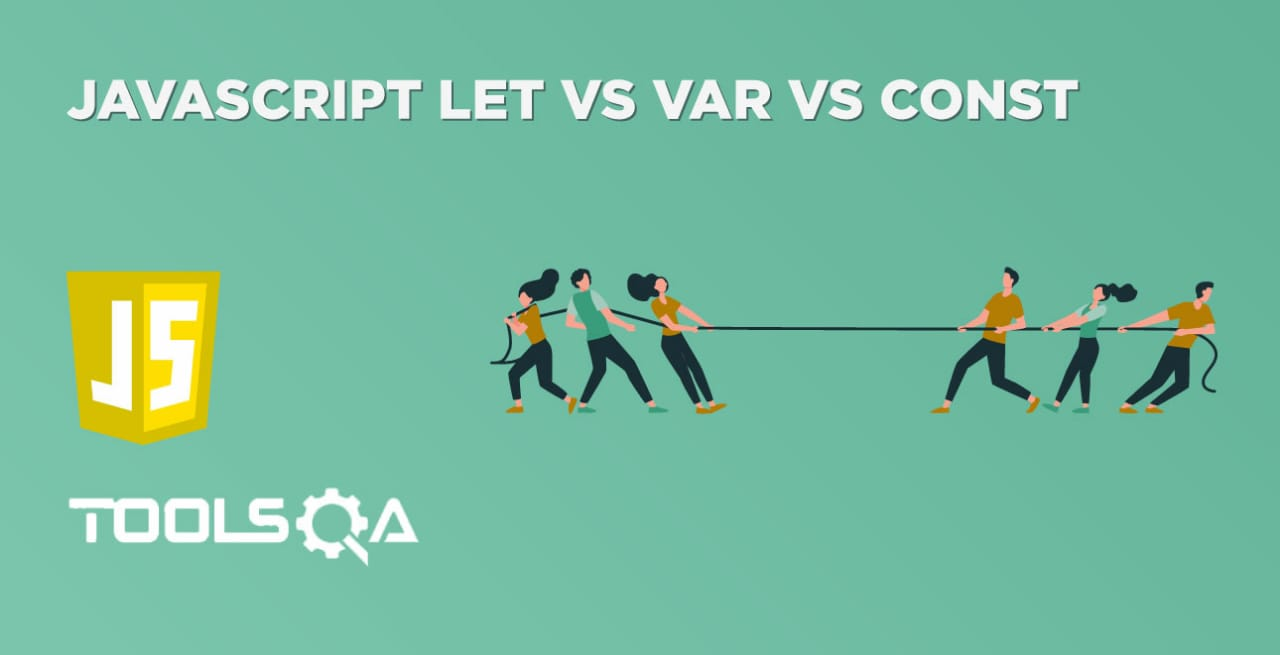Arrays in JavaScript provide multiple inbuilt functions for the manipulation of array elements. We already covered a few of them related to the addition and deletion of individual elements to the arrays in the previous article. In this article, we will extend the arrays journey and will cover details of following inbuilt Javascript Array Methods provided by the array object:

• What is the map() method, and how to use it?
• What is the reduce() method, and how to use it?
• Details of the filter() method, and how to use it?
• What is the concat() method, and how to use it?
• What is the copyWithin() method, and how to use it?
• Details about the entries() method, and how to use it?
• What is the fill() method, and how to use it?

## What is map() in Javascript Array Methods, and how to use it?

The map() method creates a new array from an existing one, applying a function to each one of the elements of the first array. Additionally, its syntax will look like below:

Syntax:

``````var variableName = [value1,value2,.....,valueN]

var output = variableName.map(callingFunction());
``````

Example: Suppose we want to return a new array from the existing array by adding 50 to each of the elements of the current array. The following example shows a code snippet of how to achieve the same using the ".map()" method:

``````<html>

<body>

Demonstrating map function in javascript.

</br>

<script type = "text/javascript">

var arrayValue  = [9,3,7,21,6,8];

var output = arrayValue.map(x => x+50);

for(i =0 ;i<output.length;i++){

document.write(output[i]+"</br>");

}

</script>

</body>

</html>

``````

Save the file with name mapFunction.html and open it in any browser (Chrome, Firefox, or IE). It should show the output asIn the above code, the map function adds 50 to each value of the array. At line 7, the map function is being invoked on the array "arrayValue" which reads each value of the array in a variable x, adds 50 to it. Consequently, the assignment of the whole array then happens to a new array named "output". In the next lines, we just printed the values of the new array, which confirms that the values in the "output" array are the values after adding 50 to each of the array elements.

### When to use the Map Method?

As we covered in previous articles, there are other array methods also, like the ForEach method to achieve the same task, you might wonder, "when should I use the map method?" Here are some questions that can help you decide:

1. Do I need to return an array from the method?
2. Am I returning a value from the callback function?

If your answer to any of these questions is Yes, you should use the map function. Otherwise, you should probably use forEach or for..of instead.

## What is reduce() in Javascript Array Methods, and how to use it?

The reduce() method reduces an array of values down to just one value. To get the output value, it runs a reducer function on each element of the array. Moreover, its syntax will look like below:

Syntax:

``````var variable = [value1, value2, value3….,valueN];
var output = variable.reduce(function);
``````

The following code snippet shows a working example of the reduce() function of Javascript Array Methods, which results in the difference of all the elements of the array starting from the first element to the last element:

``````<html>
<body>
Demonstrating reduce function in javascript.
</br>
<script type="text/javascript">
var arrayValue = [500, 200, 100, 100];
var iteration = 1;
var output = arrayValue.reduce(userFunction); // Perform reduce on each of the elements

function userFunction(firstValue, restTotalValue)
{
document.write('Running Iteration: ', iteration, '</br>');
document.write('Initial value: ', firstValue, '</br>');
document.write('Next value: ', restTotalValue, '</br>');
document.write('</br>');
iteration++;
return firstValue - restTotalValue;
}

document.write('Final Result:', output);
</script>
</body>

</html>

``````

Save the file with name reduceFunction.html and open it in any browser (Chrome, Firefox, or IE). It should show the output as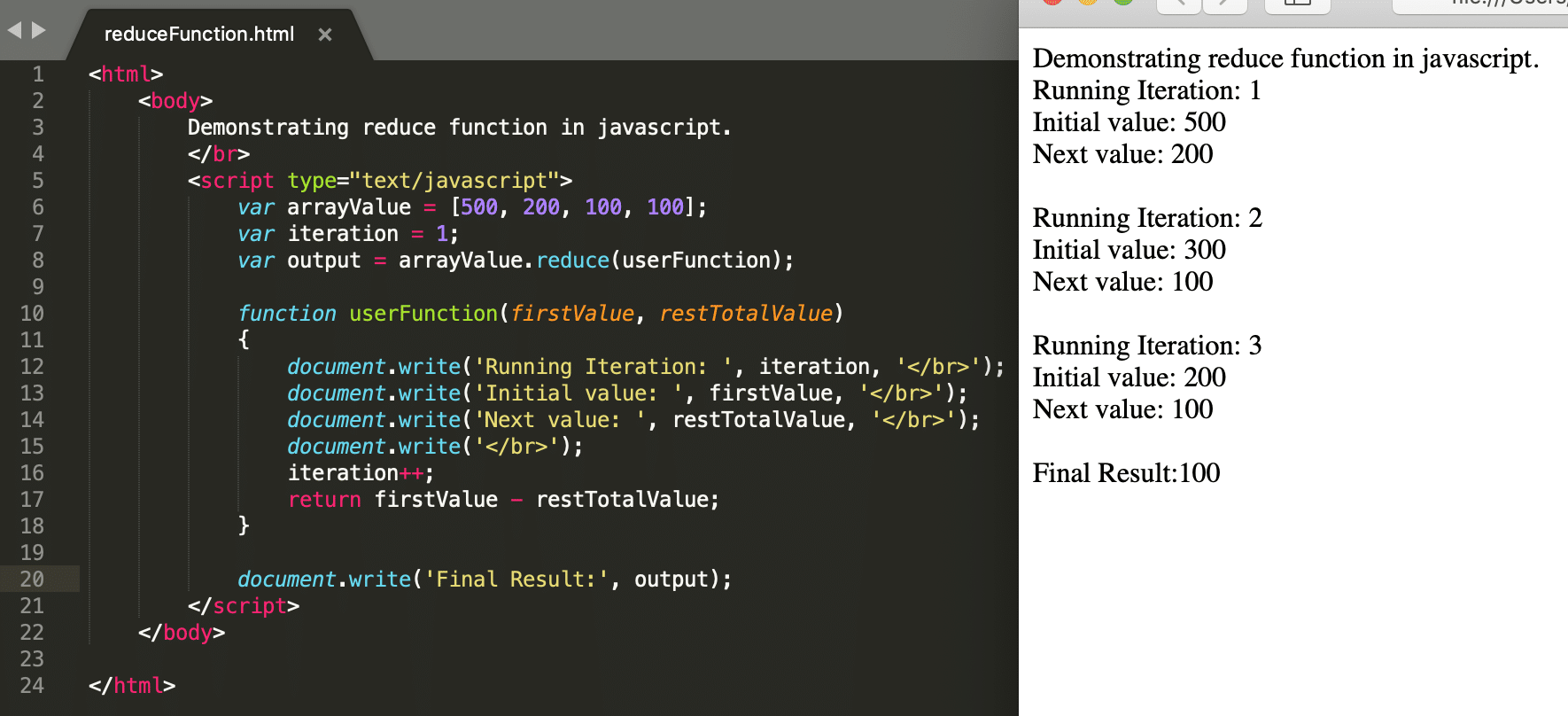It is evident from the above screenshot that the reduce() function invokes the function "userFunction()" on all the elements of the array. Moreover, the result of the first iteration becomes the initial value for the next iteration, and the next iteration performs on the result of the first iteration and the next element of the array. This way, the calculation continues until it reduces the whole array to a single element based on the logic of the function passed as a parameter.

## What is reduceRight() in Javascript Array Methods, and how to use it?

JavaScript provides one more version of the reduce() method, which works on the last element of the array, instead of the first element, and its name is "reduceRight()". Its syntax will look like below:

Syntax:

``````var variable = [value1, value2, value3….,valueN];

var output = variable.reduceRight(function);
``````

The following code snippet shows a working example of the reduceRight() function of Javascript Array Methods, which results in the difference of all the elements of the array starting from the last element:

``````<html>
<body>
Demonstrating reduceRight function in javascript.
</br>
<script type="text/javascript">
var arrayValue = [500, 200, 100, 100];
var iteration = 1;
var output = arrayValue.reduceRight(userFunction);

function userFunction(firstValue, restTotalValue)
{
document.write('Running Iteration: ', iteration, '</br>');
document.write('Initial value: ', firstValue, '</br>');
document.write('Next value: ', restTotalValue, '</br>');
document.write('</br>');
iteration++;
return firstValue - restTotalValue;
}

document.write('Final Result:', output);
</script>
</body>

</html>

``````

Save the file with name reduceRightFunction.html and open it in any browser (Chrome, Firefox, or IE). It should show the output as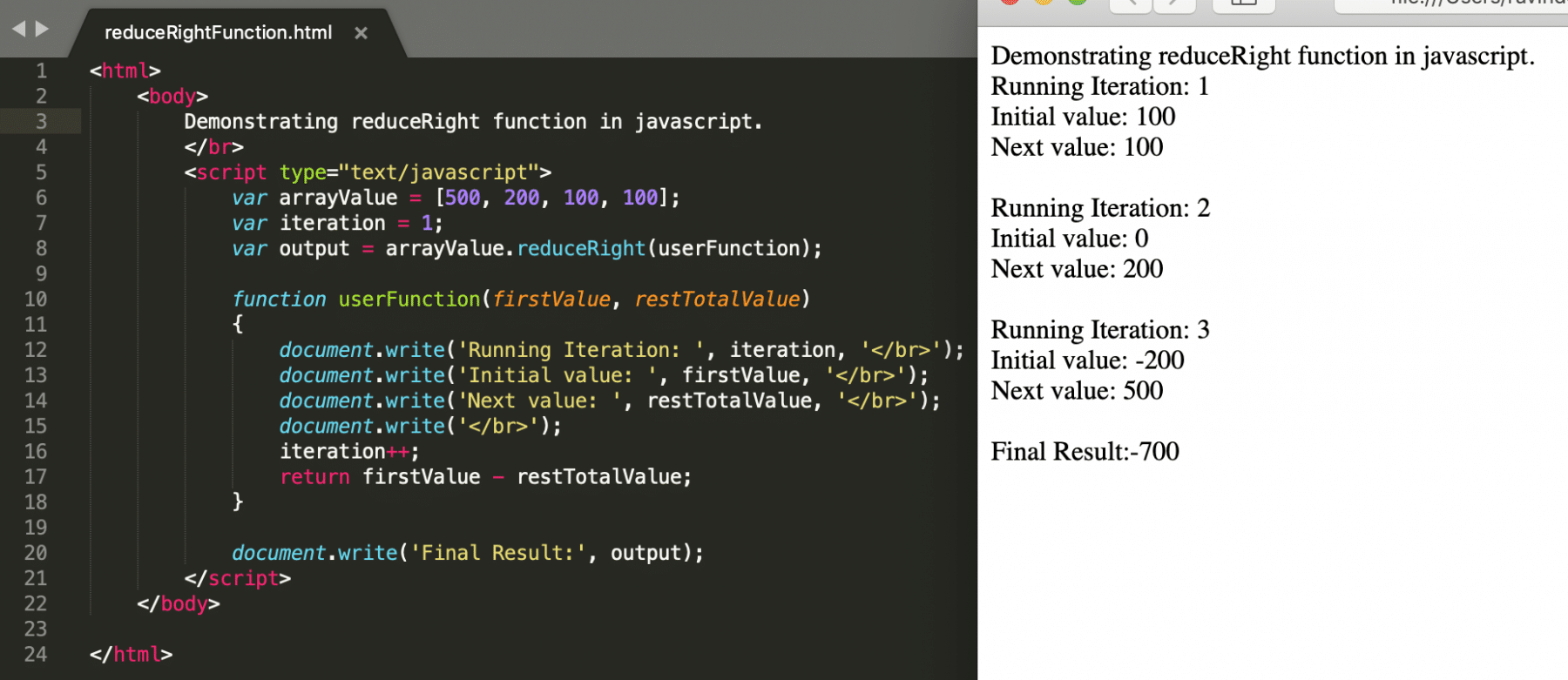It is evident from the above screenshot that the reduceRight() function invokes the callback function "userFunction()" on all the elements of the array starting from the last element. The result of the first iteration becomes the initial value for the next iteration, and the next iteration performs on the result of the first iteration and the next element of the array backward. This way, the calculation continues until it reduces the whole array to a single element based on the logic of the callback function passed as a parameter.

## What is the filter() method in JavaScript, and how to use it?

The filter() method takes each element in an array, and it applies a conditional statement against it. If this conditional statement returns true, the element gets pushed to the output array. However, if the conditional statement returns false, the element does not get pushed to the output array. Its syntax will look like below:

Syntax:

``````var variableName = [value1,value2,.....,valueN]
var output = variableName.filter(functionThatReturnsBooleanValue);
``````

The following code snippet shows a working example of the filter() function of Javascript Array Methods, which results in the difference of all the elements of the array starting from the last element:

``````<html>

<body>

Demonstrating filter function in javascript.

</br>

<script type = "text/javascript">

var arrayValue  = [2,3,4,5,6,7,8];

var output = arrayValue.filter(x => x%2==0); // Will save value to output array
// only when it is divisible by 2

for(i =0 ;i<output.length;i++){

document.write(output[i]+"</br>");

}

</script>

</body>

</html>
``````

Save the file with name filterFunction.html and open it in any browser (Chrome, Firefox, or IE). It should show the output as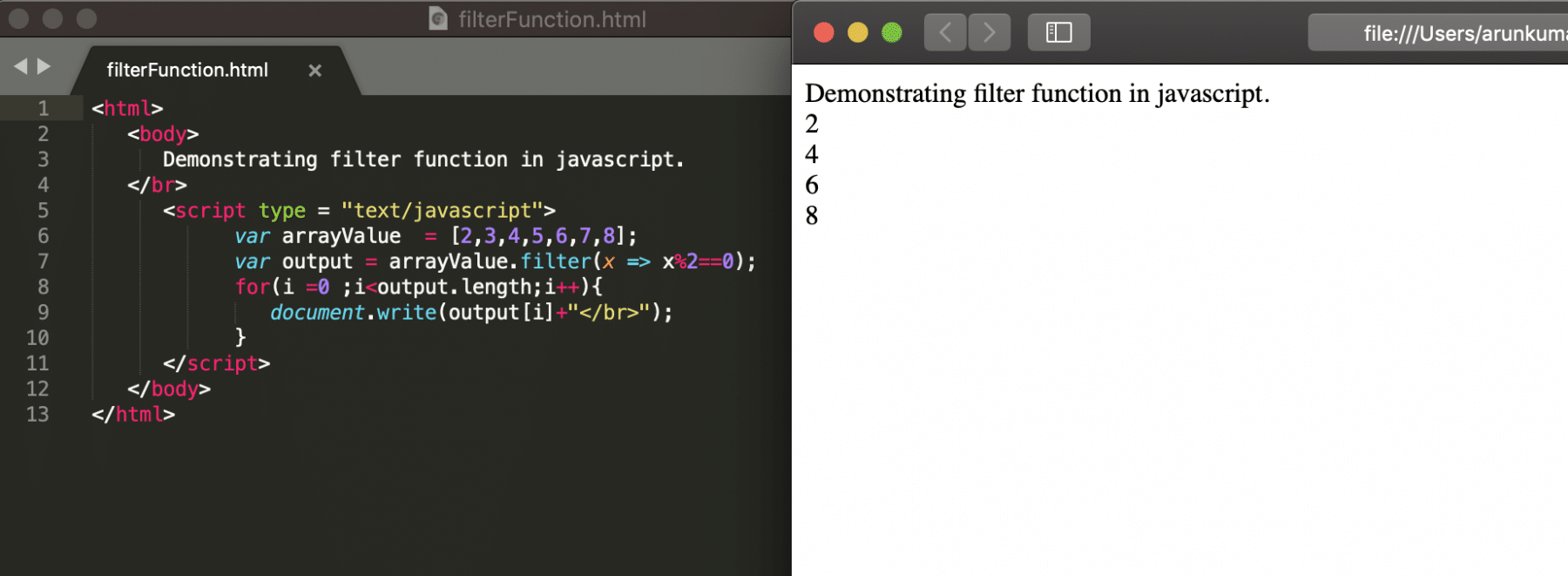The above screenshot clearly shows the output array contains the array elements which were divisible by two. Moreover, the others were being skipped from the calculation.

## What is the concat() method in JavaScript, and how to use it?

The concat() method joins or merges two or more arrays. It does not change the existing arrays but returns a new array containing the values of the joined arrays. Additionally, its syntax will look like below:

Syntax:

``````array1.concat(array2,array3,.....,arrayN);
``````

where array2, array3,.....,arrayN are the arrays that need to concatenate.

Let's see the working of concat() method with the help of following code snippet:

``````<html>
<body>
Demonstrating concat function in javascript.
</br>
<script type="text/javascript">
var array1 = [2, 3, 4, 5];
var array2 = [9, 10, 11];
var array3 = [12, 13, 14];

var output = array1.concat(array2, array3);
for (i = 0; i < output.length; i++)
{
document.write(output[i] + "</br>");
}
</script>
</body>

</html>

``````

Save the file with name concatFunction.html and open it in any browser (Chrome, Firefox, or IE). It should show the output as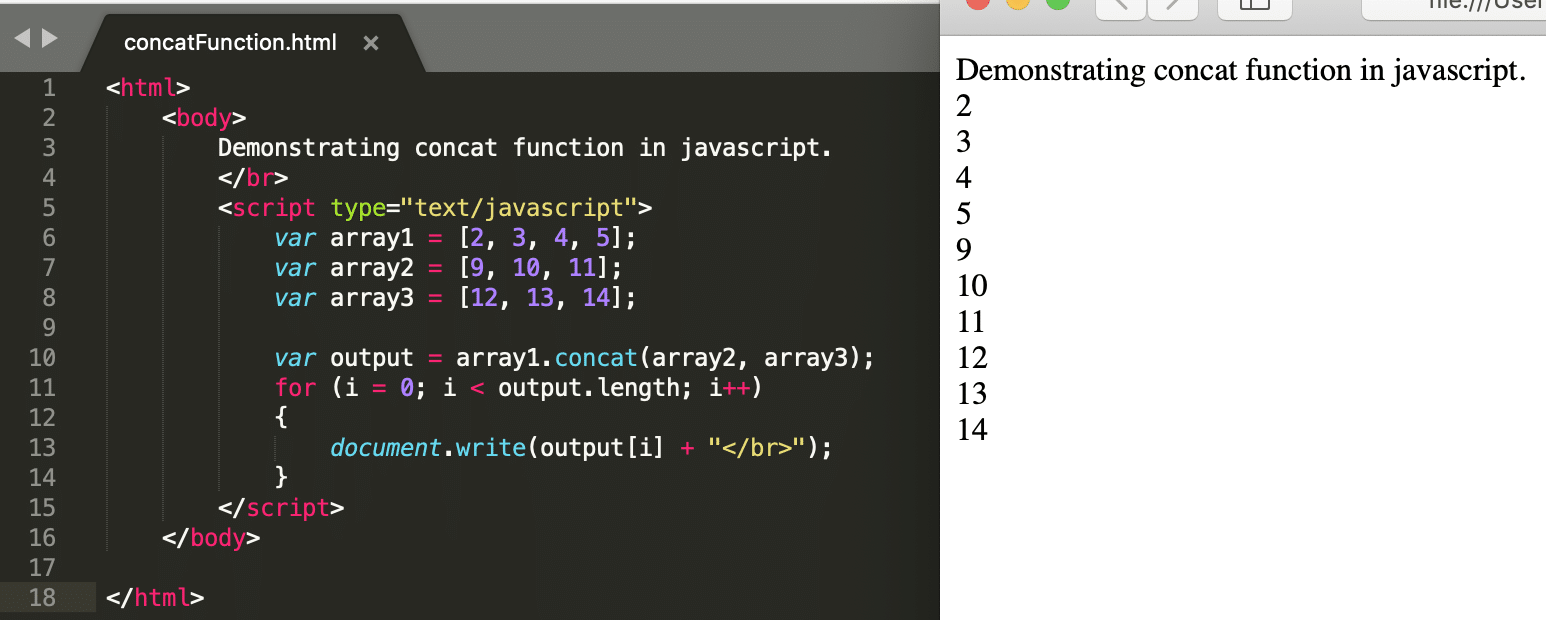The above screenshot clearly shows that the array elements of array2 and array3 merge with array1 elements, and the output array stores the result.

## What is the copyWithin() method in JavaScript, and how to use it?

This copyWithin() function copies array elements from one index to another index within the same array. By doing this, it will overwrite the existing value of that index. Additionally, its syntax will look like below:

Syntax:

``````var array1=[value1,value2,value3….,valueN];
array1.copyWithin(indexValue,startIndex,endIndex);
``````

Where:

• indexValue: the index where the copied elements need to paste.
• startIndex: The index from which copy needs to be started. By default, the value will be 0. Moreover, this field is optional.
• endIndex: The index up to which copy needs to end. By default, the value will be arraylength-1. Additionally, this field is optional.

The following code snippet shows an example demonstrating the functionality of copyWithin() method:

``````<html>
<body>
Demonstrating copyWithin function in javascript.
</br>
<script type="text/javascript">
var arrayValue = [0, 1, 2, 3, 4, 5, 6, 7, 8, 9];

// Copy elements on 6th index, starting from 0 to 2nd index,
// where 0 is inclusive and 2 is exclusive
arrayValue.copyWithin(6, 0, 2);

for (i = 0; i < arrayValue.length; i++)
{
document.write(arrayValue[i] + "</br>");
}
</script>
</body>

</html>

``````

Save the file with name copyWithinFunction.html and open it in any browser (Chrome, Firefox, or IE). It should show the output as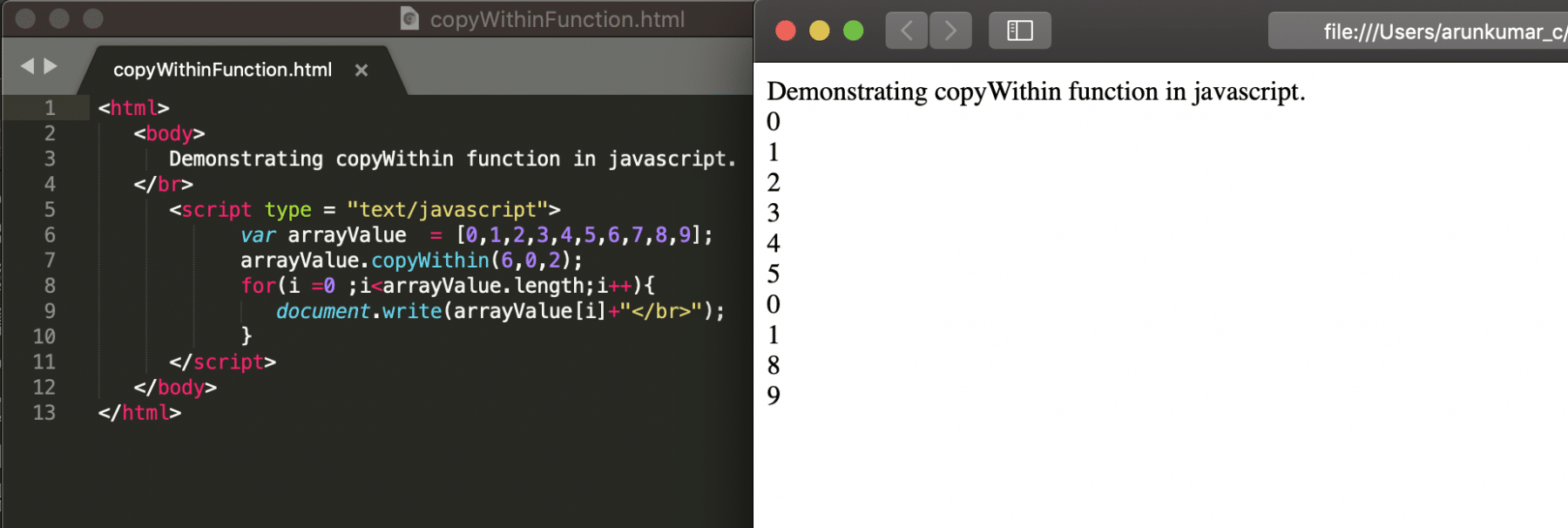The above screenshot clearly shows that 0 and 1 have replaced the 6 and 7 in the array.

## What is the entries() JavaScript function, and how to use it?

The entries() method returns an Array Iterator object with key/value pairs. For each item in the original array, the new iteration object will contain an array with the index as the key and the array item value as the value. Moreover, its syntax will look like below:

Syntax:

``````var array1=[value1,value2,value3….,valueN];
var entriedArray = array1.entries();
``````

The following code snippet shows an example of how to get all the values and indexes from an array.

``````<html>

<body>

Demonstrating entries function in javascript.

</br>

<script type = "text/javascript">

var arrayValue  = ["Tools","QA","JavaScript","Tutorial"];

var output = arrayValue.entries();

for(var x of output){

document.write(x+"</br>");

}

</script>

</body>

</html>
``````

Save the file with name entriesFunction.html and open it in any browser (Chrome, Firefox, or IE). It should show the output asThe snapshot indicates that the output variable stores all the elements of the array with their indexes as key-value pairs.

## What is the fill() JavaScript function, and how to use it?

The fill() method is used as a replace function where it will replace the element by index basis instead of value. Additionally, its syntax will look like below:

Syntax:

``````var array1=[value1,value2,value3….,valueN];
var entriedArray = array1.fill(fillValue,startIndex,EndIndex);
``````

Where,

• fillValue: The value that will replace.
• startIndex: From which, index value needs to get replaced. The default value is 0. It is an optional field.
• endIndex: Until which, index value needs to get replaced. The default value is length-1. It is an optional field.
• If there is no start end value, then all values in the array will get replaced.

The following code snippet shows an example of how to use the fill() method to replace values in an array.

``````<html>

<body>

Demonstrating fill function in javascript.

</br>

<script type = "text/javascript">

var arrayValue  = ["Tools","QA","JavaScript","Tutorial"];

var output = arrayValue.fill("Tuts",3,4);

for(var x of output){

document.write(x+"</br>");

}

</script>

</body>

</html>
``````

Save the file with name fillFunction.html and open it in any browser (Chrome, Firefox, or IE). It should show the output as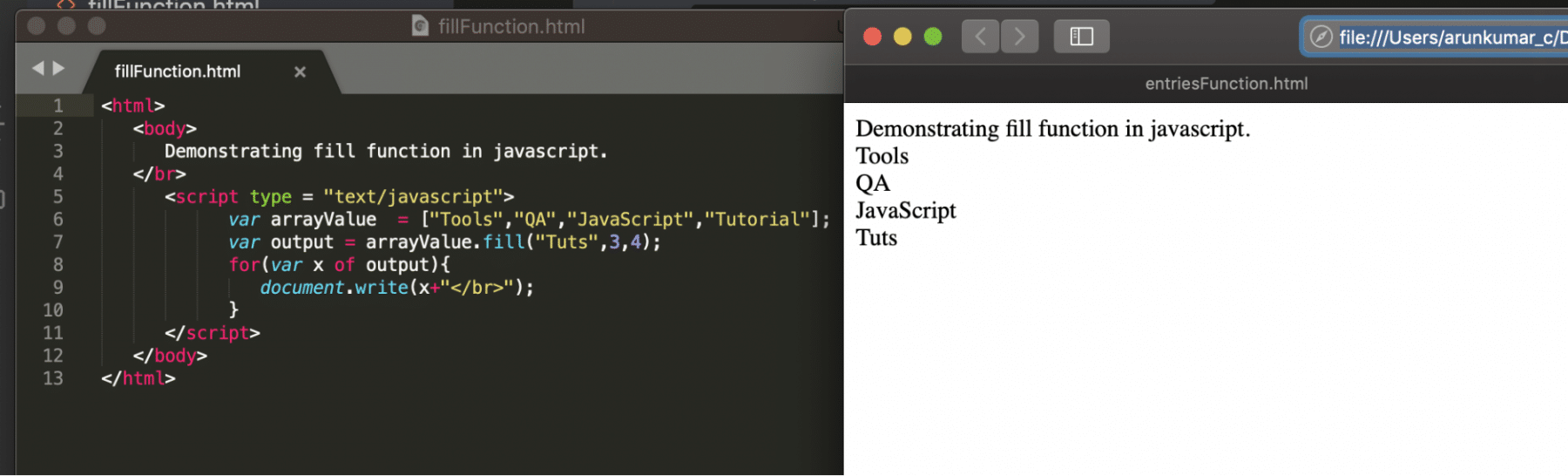The above screenshot clearly shows that the "Tuts" replaces the "Tutorials" value in the final output.

## Key Takeaways

• When you need a new array by doing some operation on all the elements of an existing array, you can use the map() method of arrays.
• When you need to reduce all the values of an array to a single element based on the logic of a function, you can use the reduce() method of arrays.
• In case you need to create an array from existing array based on a condition on the array elements, you can use the filter() method of arrays.
• When you need to merge two or more arrays, you can use the concat() method of arrays.
• When you want to create an array from existing array by copying elements between specified indices of the array, you can use the copyWithin() method of arrays.
• In case you want to get each element of the array as a key/value pair, you can use the entries() method of arrays.

To conclude, let's now move to the next article to understand what are the various ways to declare variables in JavaScript and how do they differ as per scope and usage when declared using Var, Let and Const keywords.